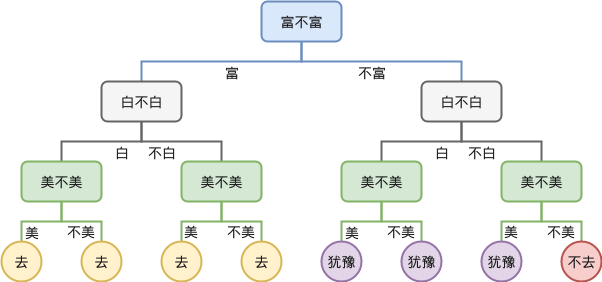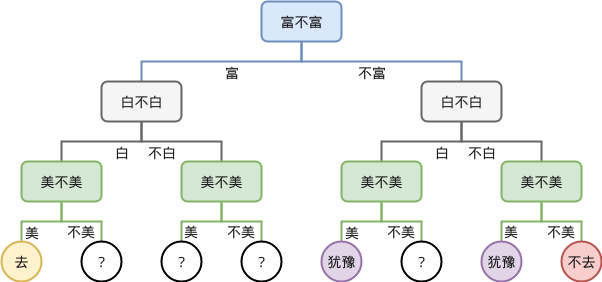1. 我们说要训练/学习，训练/学习什么？
2. 为什么决策树可以用来预测？或者说它的泛化能力的来源是哪？

## 什么是决策树？## 训练，训练什么？（当然上面的说法不准确，决策树就是一棵树，建成什么样子其实全凭心情/需求。在搜索过程中其实并找不到官方的优化目标，上面的结论是博主自己得出的，而它能帮助我们理解下一个问题：决策树为什么有泛化能力？）

## 泛化能力

### 节点的合并是泛化能力的根本## 贪心指标

### 基尼不纯度 Gini impurity

$$I_{G}( p ) = \sum_{i=1}^J p_i \sum_{k\neq i} p_k = \sum_{i=1}^{J} p_i (1-p_i) = \sum_{i=1}^{J} (p_i - {p_i}^2) = \sum_{i=1}^J p_i - \sum_{i=1}^{J} {p_i}^2 = 1 - \sum^{J}_{i=1} {p_i}^{2}$$

### 信息熵增益 Information Gain

$$H(X) = \sum_{i}{P(x_i)I(x_i)} = -\sum_i{P(x_i)\log_2{P(x_i)}}$$

$-\log_2{P(x_i)}$ 的大意是一个事件 $x_i$ 如果出现的概率越小，那么当它发生时我们就越吃惊，代表的就是一个事件“吃惊程度”。而熵就是所有事件的“吃惊程度”的期望值。一般地，如果一个集合的熵越大，则集合越无序；熵越小，则集合越有序。换句话说，如果熵越大，说明我们越容易吃惊，说明集合无序，我们很难预测下一个出现的是什么，相反，熵越小，说明我们越容易猜测集合里有什么，说明集合越有序。

$$H(X|Y) = \sum_{y \in Y}{p(y) H(X|Y=y)}$$

$$Gain(Y) = H(X) - H(X|Y)$$

## 决策树的实现

_get_feature，我们按输入的维度顺序切分，因此实现是：

## 小结

“训练”决策树是为了减少决策树最后的叶子节点，由于训练全局最优很困难，因此人们用一些局部的贪心策略进行训练，例如上文介绍的信息熵增益。